# Circuit Analysis II

This is the second semester of a beginning circuit analysis course.

# Chapter 1 Thévenin’s and Norton's Theorems

## Thévenin’s Theorem Review

In this chapter, we will cover the following topics:

•  Thévenin’s Theorem

•  Finding Thévenin’s equivalents

•  Example of finding a Thévenin’s equivalent

### Thévenin’s Theorem Defined

Thévenin’s Theorem is another equivalent circuit. Thévenin’s Theorem can be stated as follows:

Any circuit made up of resistors and sources, viewed from two terminals of that circuit, is equivalent to a voltage source in series with a resistance.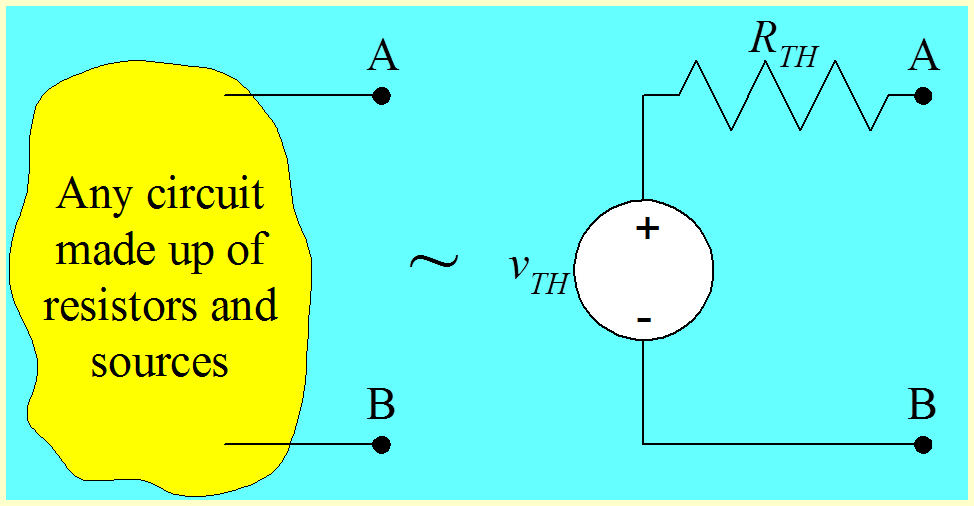Figure 1.1 Thévenin Equivalent. Courtesy of David Shattuck, used with personal permission.

The voltage source is equal to the open-circuit voltage for the
two-terminal circuit, and the
resistance is equal to the
equivalent resistance of the circuit.  We can write this in equation form as

We have introduced a term called the open-circuit voltage. This is the voltage for the circuit that we are finding the equivalent of, with nothing connected to the circuit.  Connecting nothing means an open circuit.  This voltage is shown here, with the voltage vOC being defined at the terminals of the yellow circuit, with nothing being added.

The polarities of the source with respect to the terminals is important. If the reference polarity for the open-circuit voltage is as given here (voltage drop from A to B), then the reference polarity for the voltage source must be as given here at the right of Figure 1.2 (positive terminal closer to A, and negative terminal closer to B).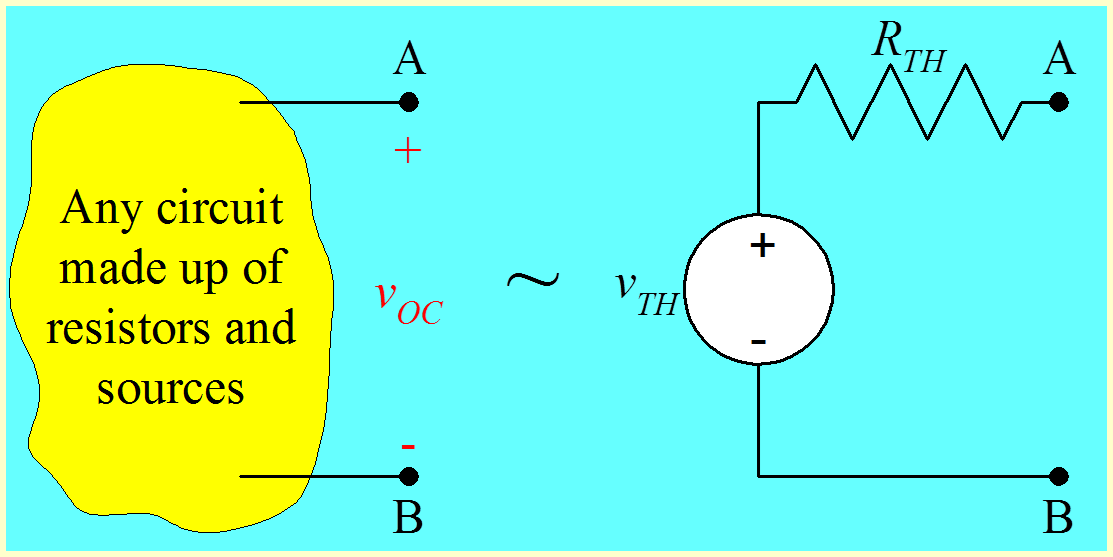Figure 1.2 Definition of the Open Circuit Voltage. Courtesy of David Shattuck, used with personal permission.

We have introduced a term called the equivalent resistance. This is the resistance for the circuit that we are finding the equivalent of, with the independent sources set equal to zero. Any dependent sources are left in place.

As with all equivalent circuits, these two are equivalent only with respect to the things connected to the equivalent circuits.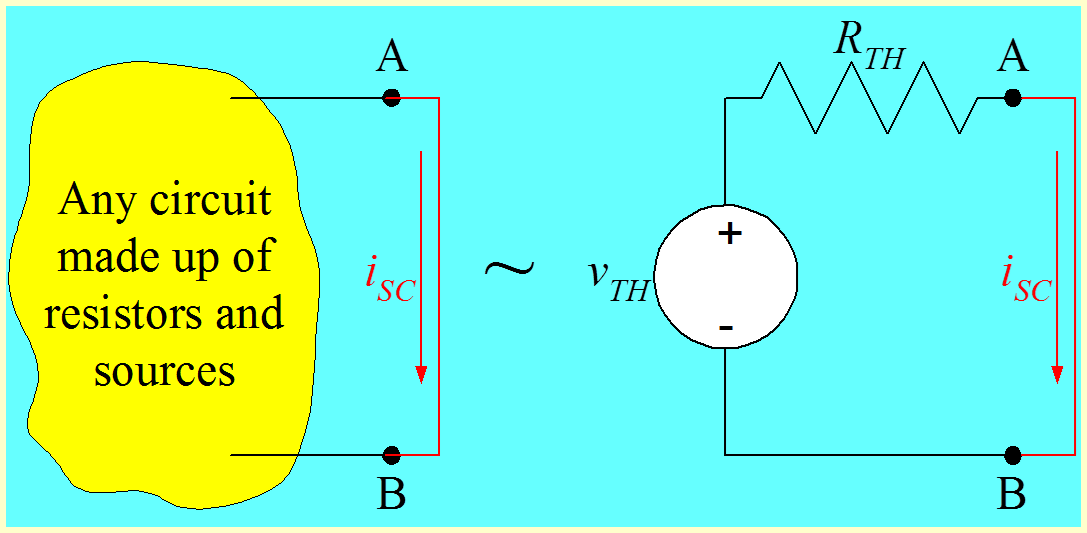Figure 1.3 Definition of the Short Circuit Current. Courtesy of David Shattuck, used with personal permission.

A useful concept is the concept of short-circuit current. This is the current that flows through a wire, or short circuit, connected to the terminals of the circuit. This current is shown here as iSC.

The polarities of the source with respect to the terminals is important. If the reference polarity for the short-circuit current is as given here (flowing through the wire from A to B), then the reference polarity for the voltage source must be as given here (positive terminal closer to A, and negative terminal closer to B), where we are relating these three quantities with the equation,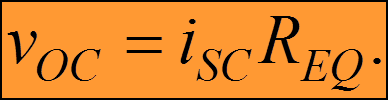Equation 1.3 This is not Ohm's Law, but it does look just like Ohm's Law.

When we look at the circuit on the right of Figure 1.3, we can see that the short-circuit current is equal to vTH/RTH, which is also vOC/REQ. Thus, we obtain the important expression for iSC, shown here in Equation 1.3.

Not Ohm's Law?Why do we say that this equation is not Ohm's Law? Why not?

Note:  You will note be credited with getting the correct answer unless your exact wording is the same as mine.  This is not important, since credit is being given for participation here.  We will discuss the correct answer in class later.

### Any Two of the Three Quantities

For the Thévenin equivalent, the open-circuit voltage is equal to the short-circuit current times the equivalent resistance. In general we can find the Thévenin equivalent of a circuit by finding
any two of the following three things in the equation

The three things are

1) the open circuit voltage, vOC

2) the short-circuit current, iSC, and

3) the equivalent resistance, REQ

Once we find any two, we can find the third by using this equation.

One more time, the reference polarities of our voltages and currents matter. If we pick vOC at A with respect to B, then we need to pick iSC going from A to B. If not, we need to change the sign in this equation.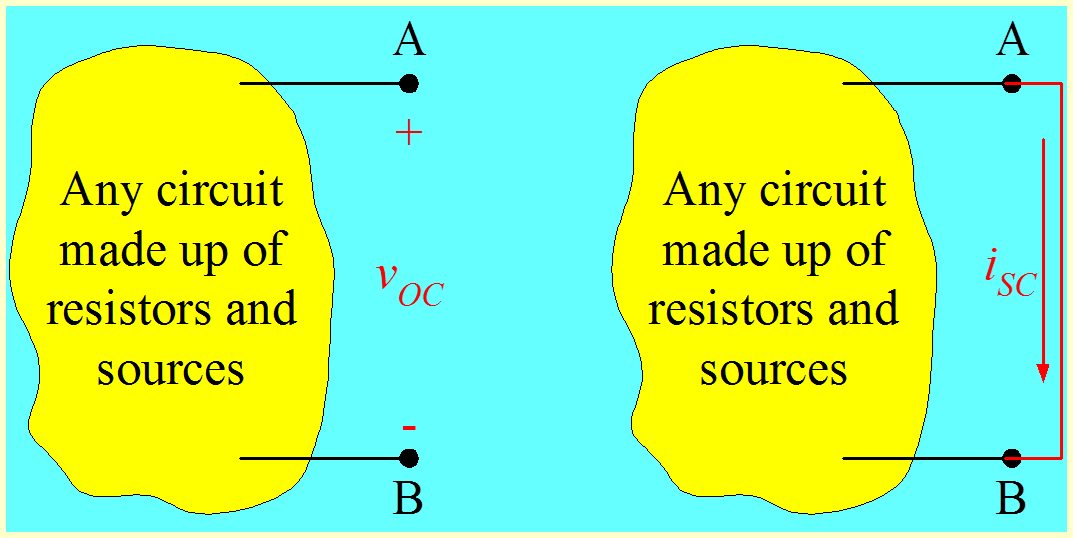Figure 1.4 Relationship of vOC and iSC. Courtesy of David Shattuck, used with personal permission.

As an example, if we pick vOC and iSC with the reference polarities given here, we need to change the sign in the equation as shown.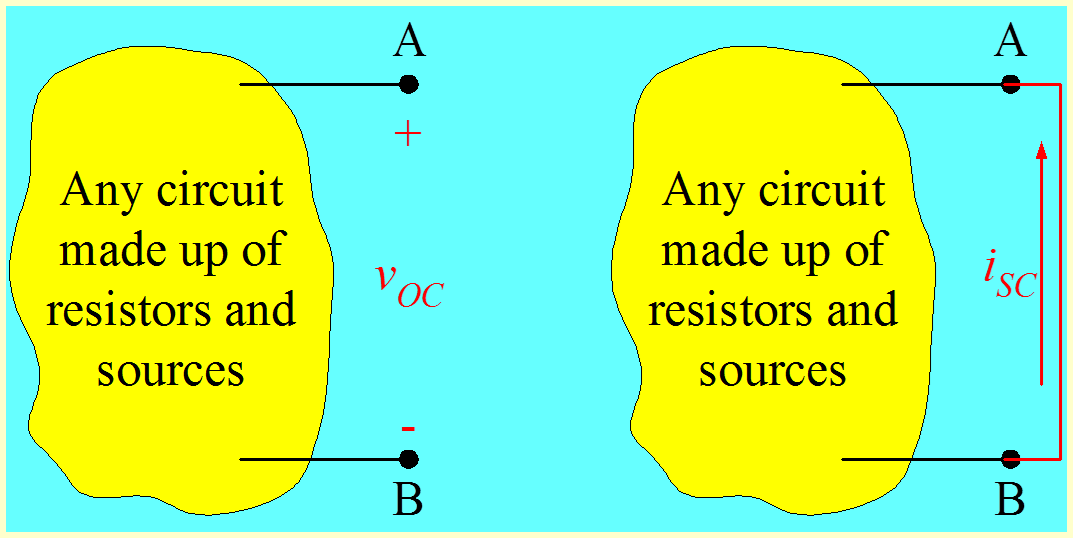Figure 1.5 Alternative relationship of vOC and iSC. Courtesy of David Shattuck, used with personal permission.

This is a consequence of the sign in Ohm’s Law. For a further explanation, see the next diagram.  Note that in this diagram, where the short circuit current iSC is defined going from B to A through the short circuit, that current is flowing through the resistance REQ in active sign relationship with respect to the voltage vOC which is set by the polarity of the voltage source, in turn set by the choice of definition of the open circuit voltage at A with respect to B.

Be very careful here! We have labeled the voltage across the resistance REQ as vOC. This is true only for this special case. This vOC is not the voltage at A with respect to B in this circuit. In this circuit, that voltage is zero due to the short. Due to the short, the voltage across REQ is vOC.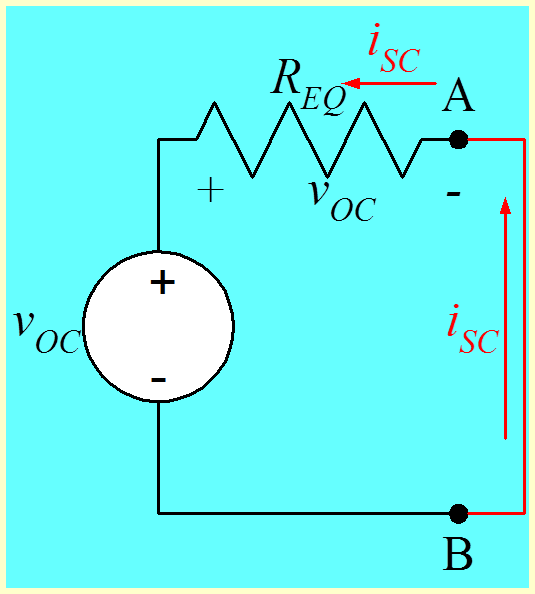Figure 1.6 Alternative relationship of vOC and iSC. Courtesy of David Shattuck, used with personal permission.

Ohm's LawWait a minute. We said before it was not Ohm's Law, but now we say it is. What is going on here?

A

It was always, and is always, Ohm's Law.

B

The equation as given relates the voltage in one circuit, with the current in another circuit. As such it is not Ohm's Law. However, it comes from Ohm's Law in a particular situation.

C

Sometimes it is Ohm's Law, and sometimes it is not.

D

All of the above.

When we have dependent sources in the circuit in yellow, it will make some calculations more difficult, but does not change the validity of the theorem.

### Summary to this Point

1.  We can find the Thévenin equivalent of any circuit made up of voltage sources, current sources, and resistors. The sources can be any combination of dependent and independent sources.

2. We can find the values of the Thévenin equivalent by finding the open-circuit voltage and short-circuit current. The reference polarities of these quantities are important.

3. To find the equivalent resistance, we need to set the independent sources equal to zero. However, the dependent sources will remain. This requires some care. We will discuss finding the equivalent resistance with dependent sources in a later part of this section.

4. As with all equivalent circuits, the Thévenin equivalent is equivalent only with respect to the things connected to it.

### Example Problem 1.1

We wish to find the Thévenin equivalent of the circuit below, as seen from terminals A and B.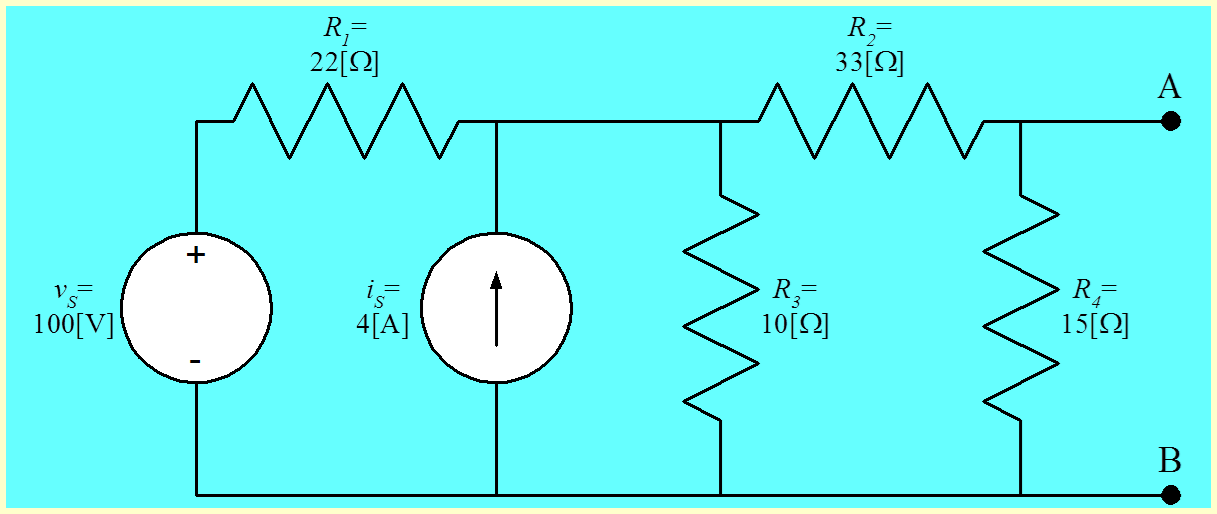Figure 1.7 Circuit for Example 1.1. Note that there is an unstated assumption here; we assume that we will later connect something to these two terminals. Having found the Thévenin equivalent, we will be able to solve that circuit more easily by using that equivalent. Courtesy of David Shattuck, used with personal permission.

We wish to find the open-circuit voltage vOC with the polarity defined in the circuit given below. We have also defined the node voltage vC, which we will use to find vOC.

In general, remember, we need to find two out of three of the quantities vOC, iSC, and REQ. In this problem we will find two, and then find the third just as a check. In general, finding the third quantity is not required.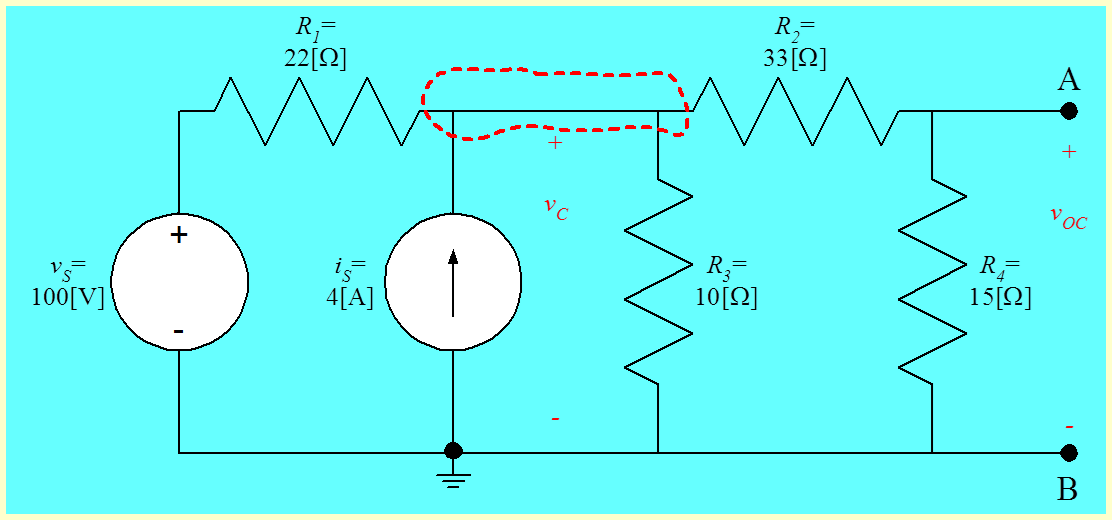Figure 1.8 Open-Circuit Voltage Circuit for Example 1.1. Courtesy of David Shattuck, used with personal permission.

Writing KCL at the node encircled with a dashed red line, we have

Plugging in values, we get

Then, using VDR, we can find

Next, we will find the equivalent resistance, REQ. The first step in this solution is to set the independent sources equal to zero. We then have the circuit below.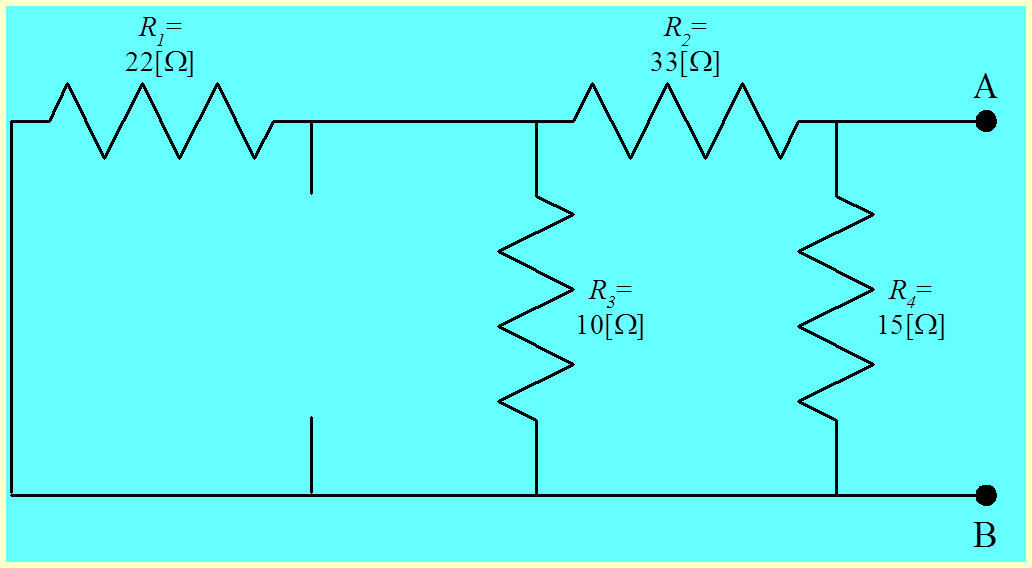Figure 1.9 Circuit for Equivalent Resistance. Note that the voltage source becomes a short circuit, and the current source becomes an open circuit. These represent zero-valued sources. Courtesy of David Shattuck, used with personal permission.

To find the equivalent resistance, REQ, we simply combine resistances in parallel and in series. The resistance between terminals A and B, which we are calling REQ, is found be recognizing that R1 and R3 are in parallel. That parallel combination is in series with R2. That series combination is in parallel with R4. We have

To complete this problem, we would typically redraw the circuit, showing the complete Thévenin’s equivalent, along with terminals A and B. This has been done here. This shows the proper polarity for the voltage source.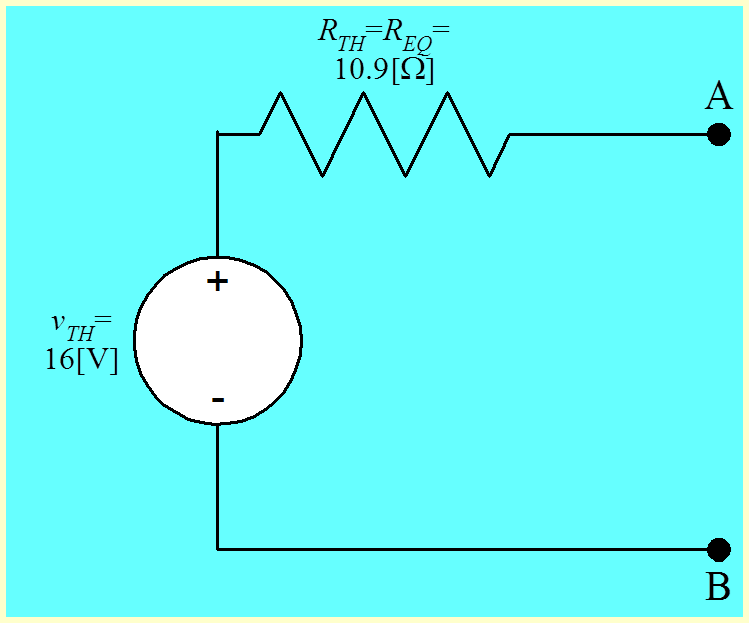Figure 1.10 Solution for Example Problem 1.1. Because we solved for the voltage at A with respect to B, the value is attached to the voltage source with the plus sign closer to A, and the minus sign closer to B. Courtesy of David Shattuck, used with personal permission.

Let’s check this solution, by finding the short-circuit current in the original circuit, and compare it to the short-circuit current in the Thévenin’s equivalent. We will start with the Thévenin’s equivalent shown here. We have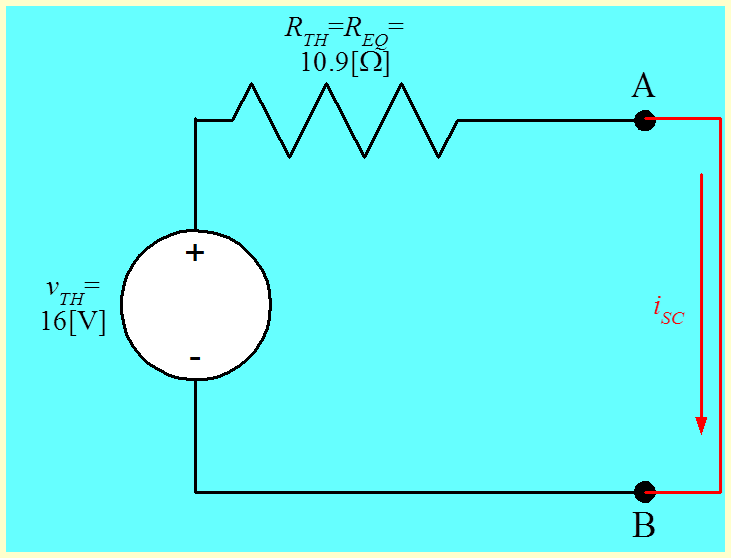Figure 1.11 Testing the Solution for Example Problem 1.1. Courtesy of David Shattuck, used with personal permission.

Let’s find the short-circuit current in the original circuit.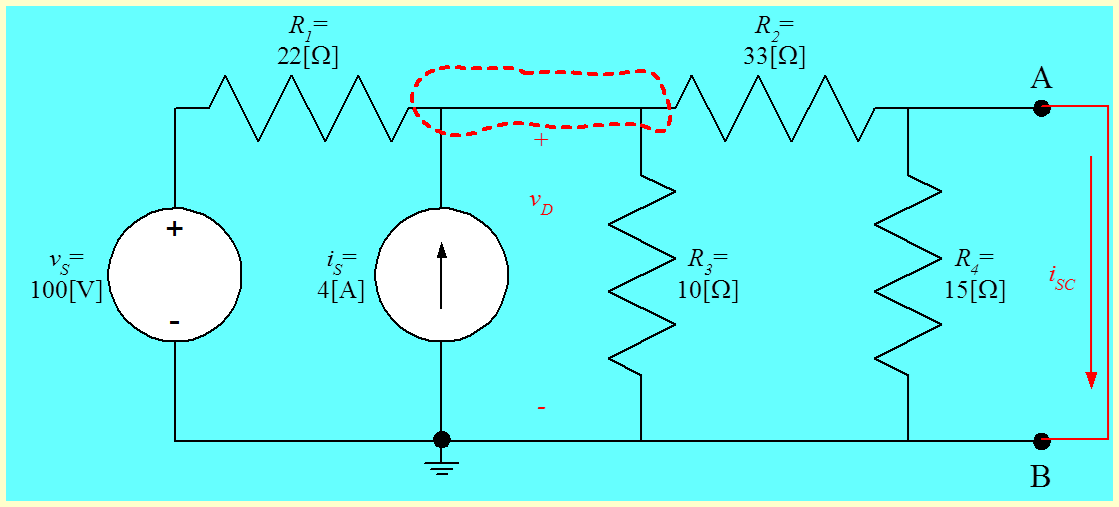Figure 1.12 Testing the Solution for Example Problem 1.1, in a Different Way. Courtesy of David Shattuck, used with personal permission.

We have KCL at the dashed closed surface,

Note that resistor R4 is neglected, since it has no voltage across it, and therefore no current through it.   With this result, we can find the short-circuit current in the original circuit,

This is the same result that we found using the Thévenin’s equivalent earlier.  The solution checks.  This is important. This shows that we could indeed have found any two of three of the quantities: open-circuit voltage, short-circuit current, and equivalent resistance, as we can see from

### Summary

What is the deal here?  Is this worth all this trouble?   This is a good question. The deal here is that Thévenin’s Theorem is a very big deal. It is difficult to convey the full power of it at this stage in your education. However, you may be able to imagine that it is very useful to be able to take a very complicated circuit, and replace it with a pretty simple circuit. In many cases, it is very definitely worth all this trouble.  There is one example you may have seen in electronics laboratories. There, the signal generator outputs are typically labeled 50[$\Omega$].  This means that the Thévenin’s equivalent resistance, for the complicated circuit inside the generator, is 50[$\Omega$], as viewed from the output terminals.  Knowing this makes using the generator easier. We view the generator as just an adjustable voltage source in series with a 50[$\Omega$] resistor.

## Norton's Theorem

In this part, we will cover the following topics:

•  Norton’s Theorem Defined
•  Finding Norton’s equivalents
•  Example of finding a Norton’s equivalent

### Norton’s Theorem Defined

Norton’s Theorem is another equivalent circuit. Norton’s Theorem can be stated as follows:  Any circuit made up of resistors and sources, viewed from two terminals of that circuit, is equivalent to a current source in parallel with a resistance.

The current source is equal to the short-circuit current for the two-terminal circuit, and the resistance is equal to the equivalent resistance of the circuit.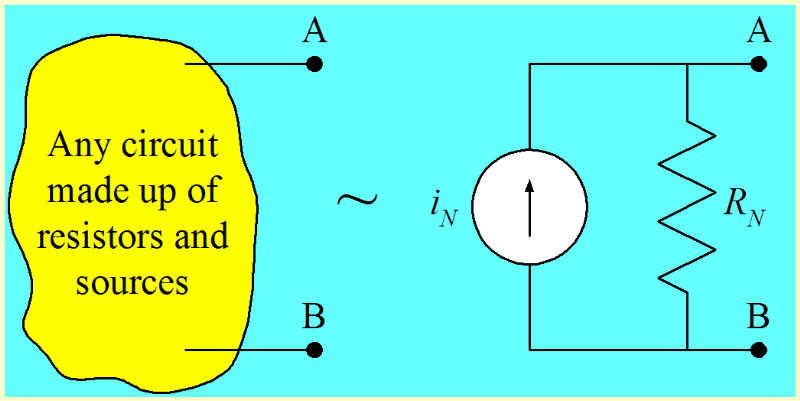Figure 1.13 Norton's Theorem. Courtesy of David Shattuck, used with personal permission.

This holds for when

It is probably obvious to you, if you studied the last parts of this chapter, that if Thévenin’s Theorem is valid, then Norton’s Theorem is valid, because Norton’s Theorem is simply a source transformation of Thévenin’s Theorem. Note that the resistance value is the same in both cases, that is, RTH = RN = REQ.  We remember that

The polarities for sources are almost always important.  Here the polarity of the current source depends on the reference polarity of the short-circuit current.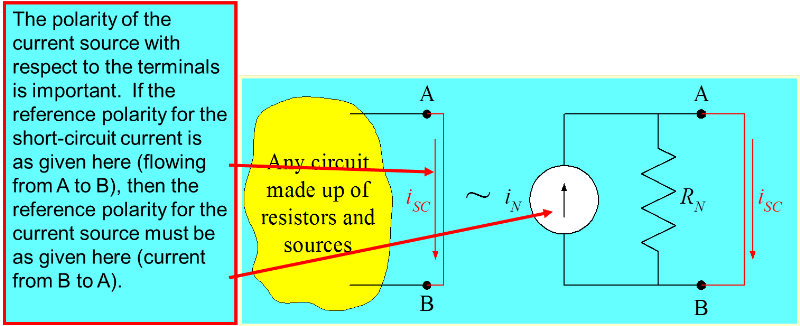Figure 1.14 Polarity of Current Source. Courtesy of David Shattuck, used with personal permission.

As with all equivalent circuits, these two are equivalent only with respect to the things connected to the equivalent circuits.  When we have dependent sources in the circuit shown here, it will make some calculations more difficult, but does not change the validity of the theorem.  The open-circuit voltage that results from the Norton equivalent is equal to the product of the Norton current source and the Norton resistance. This leads to the same equation that we used previously, for the Thévenin equivalent,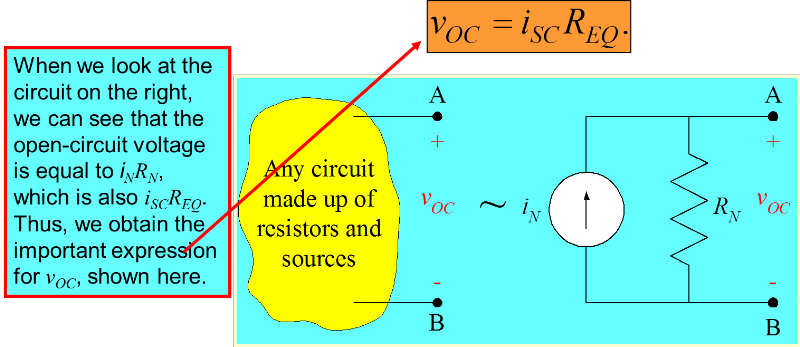Figure 1.15 Equation for the Open-Circuit Voltage. Courtesy of David Shattuck, used with personal permission.

We have shown that for the Norton equivalent, the open-circuit voltage is equal to the short-circuit current times the equivalent resistance. This is fundamental and important. However, it is not Ohm’s Law.  Remember that iSC = iN, and REQ = RN.  This was given earlier in Equation 1.13.  In general we can find the Norton equivalent of a circuit by finding any two of the following three things:

1)  the open circuit voltage, vOC,
2)  the short-circuit current, iSC, and
3)  the equivalent resistance, REQ.

As before, in Thevenin's theorem, we have to be careful about our choices of reference polarities for vOC and iSC.  This is explained in the following figures.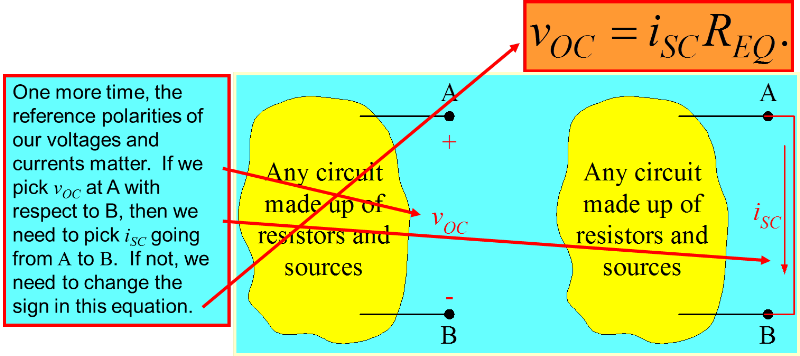Figure 1.16 Sign of the Equation in Relation to Reference Polarities. Courtesy of David Shattuck, used with personal permission.

The alternative option is shown in the next figure.

As an example, if we pick vOC and iSC with the reference polarities given here, we need to change the sign in the equation as shown. This is a consequence of Ohm’s Law, which for resistor RN requires a minus sign, since the voltage and current are in the active sign relationship in the next figure, for the resistance RN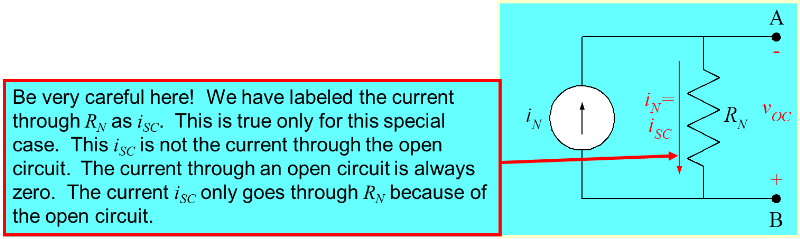Figure 1.18 Explanation for Sign Used. Courtesy of David Shattuck, used with personal permission.

### Summary

1.  We can find the Norton equivalent of any circuit made up of voltage sources, current sources, and resistors. The sources can be any combination of dependent and independent sources.

2.  We can find the values of the Norton equivalent by finding the open-circuit voltage and short-circuit current. The reference polarities of these quantities are important.

3.  To find the equivalent resistance, we need to set the independent sources equal to zero. However, the dependent sources will remain. This requires some care. We will discuss finding the equivalent resistance with dependent sources in the next part of this chapter.

4.  As with all equivalent circuits, the Norton equivalent is equivalent only with respect to the things connected to it.

What is the deal here?  Is this worth all this trouble?   This is a good question. The deal here is that Norton’s Theorem is a very big deal. It is difficult to convey the full power of it at this stage in your education. However, you may be able to imagine that it is very useful to be able to take a very complicated circuit, and replace it with a pretty simple circuit. In many cases, it is very definitely worth all this trouble.  Norton’s Theorem is often treated as being less important than Thévenin’s Theorem.  Actually, they are of equal importance, since they say essentially the same thing.  One is no more important than the other.

### Example Problem 1.2

We wish to find the Norton equivalent of the circuit below, as seen from terminals A and B.  Note that there is an unstated assumption here; we assume that we will later connect something to these two terminals. Having found the Norton equivalent, we will be able to solve that circuit more easily by using that equivalent.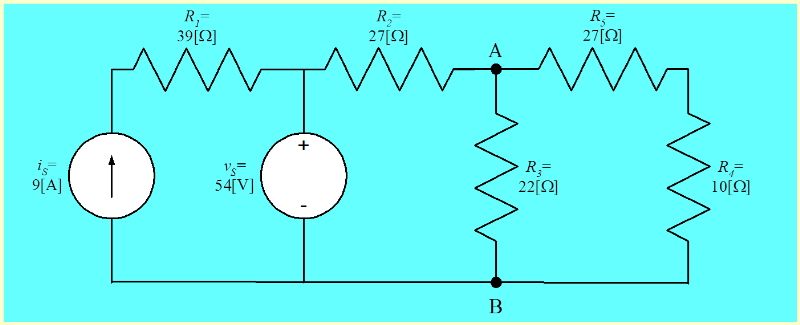Figure 1.19 Circuit for Example Problem 1.2. Courtesy of David Shattuck, used with personal permission.

We wish to find the open-circuit voltage vOC with the polarity defined in the circuit given below.  In general, remember, we need to find two out of three of the quantities vOC, iSC, and REQ. In this problem we will find two, and then find the third just as a check. In general, finding the third quantity is not required.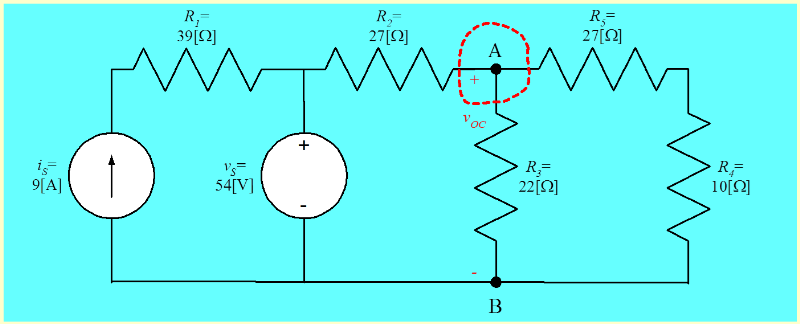Figure 1.20 Defining vOC for this Circuit. Courtesy of David Shattuck, used with personal permission.

Some students may be tempted to remove resistor R3 from this circuit. We should not do this. In future problems, if we are asked to find “the equivalent circuit seen by resistor R3”, then we assume that the resistor “does not see itself”, and remove it. In this problem, we are not given this instruction. Leave the resistor in place, even though the open-circuit voltage is across it.

We wish to find the voltage vOC. Writing KCL at the node encircled with a dashed red line, we have

Substituting in values, we have

Next, we will find the equivalent resistance, REQ. The first step in this solution is to set the independent sources equal to zero. We then have the circuit below.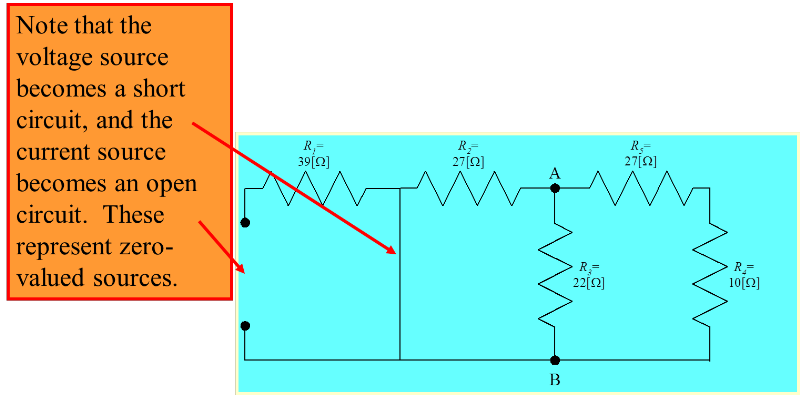Figure 1.21 Find the Equivalent Resistance, REQ. Courtesy of David Shattuck, used with personal permission.

To find the equivalent resistance, REQ, we simply combine resistances in parallel and in series. The resistance between terminals A and B, which we are calling REQ, is found by recognizing that R5 and R4 are in series. That series combination is in parallel with R2. That parallel combination is in parallel with R3. We have

Some students may have difficulty getting this expression. Remember that we are finding the resistance seen at these two terminals, A and B. The idea is that we would have this resistance if you connected a source to these two terminals.

To complete this problem, we would typically redraw the circuit, showing the complete Norton’s equivalent, along with terminals A and B. This has been done here. To get this, we need to use our equation to get the Norton current,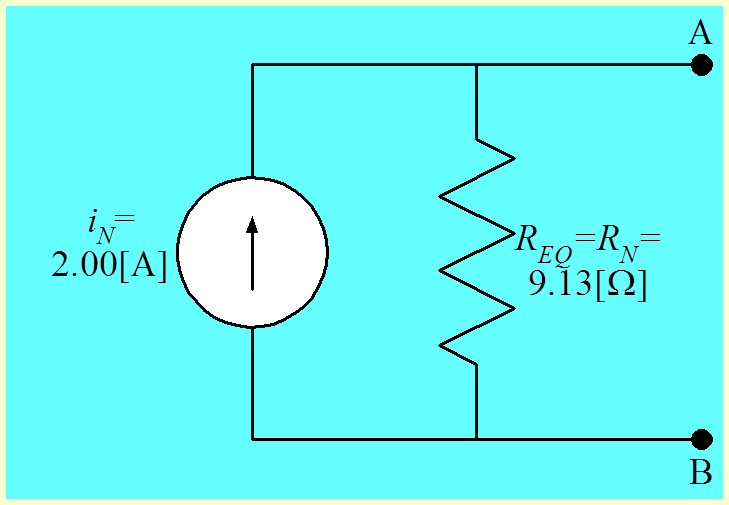Figure 1.22 Solution for Example Problem 1.2. Courtesy of David Shattuck, used with personal permission.

Let us check this solution, by finding the short-circuit current in the original circuit, and compare it to the short-circuit current in the Norton’s equivalent. We redraw the original circuit, with the short circuit current shown. We wish to find this short circuit current, iSC.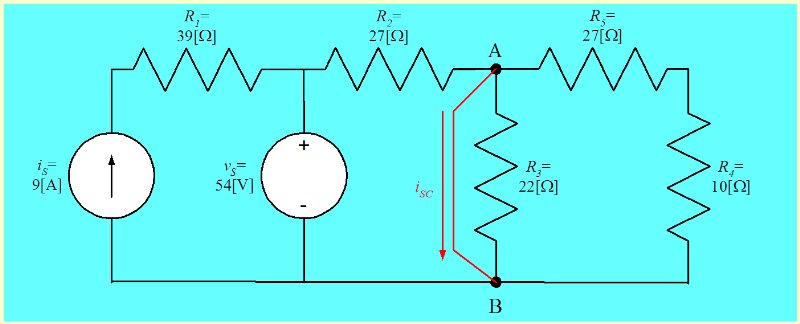Figure 1.23 Testing the Solution, Using iSC. Courtesy of David Shattuck, used with personal permission.

We start by noting that that there is no current through resistor R3, since there is no voltage across it. Another way of saying this is that the resistor R3 is in parallel with a short circuit. The parallel combination of the resistor and the short circuit, will be a short circuit.  The same exact argument can be made for the series combination of R5 and R4. This series combination is in parallel with a short circuit. Thus, we can simplify this circuit to the circuit in the next figure.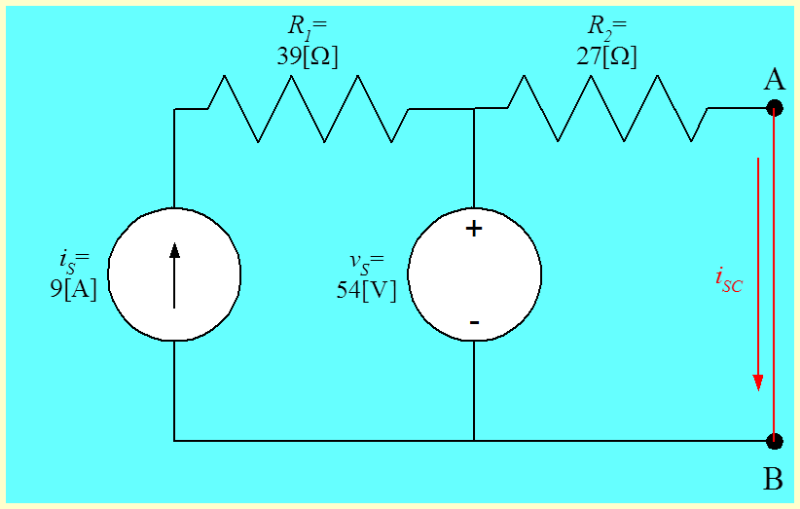Figure 1.24 Simplified Circuit for iSC. Courtesy of David Shattuck, used with personal permission.

Here, we have removed resistors R3, R4 and R5 since they do not affect the short circuit current, iSC. When we look at this circuit, we note that the voltage source vS is directly across the resistor R2, and so we can write directly,

This short-circuit current is the same result that we found in the Norton’s Equivalent earlier.  In retrospect, it is now clear that we did not take the best possible approach to this solution. If we had solved for the short-circuit current, and the equivalent resistance, we would have gotten the solution more quickly and more easily.  One of our goals is to be so good at circuit analysis that we can see ahead of time which approach will be the best for a given problem.

## Thévenin’s and Norton’s Theorems including Dependent Sources

In this part, we will cover the following topics:

• Dependent Sources and Equivalent Resistance
• The Test-Source Method
• Example of finding an equivalent resistance with a dependent source present
• Thévenin’s and Norton’s Theorems Reviewed

Thévenin’s Theorem and Norton’s Theorem can be stated as follows:

Any circuit made up of resistors and sources, viewed from two terminals of that circuit, is equivalent to a voltage source in series with a resistance, or to a current source in parallel with a resistance.

The voltage source is equal to the open-circuit voltage for the two-terminal circuit, the current source is equal to the short-circuit current for that circuit, and the resistance is equal to the equivalent resistance of that circuit.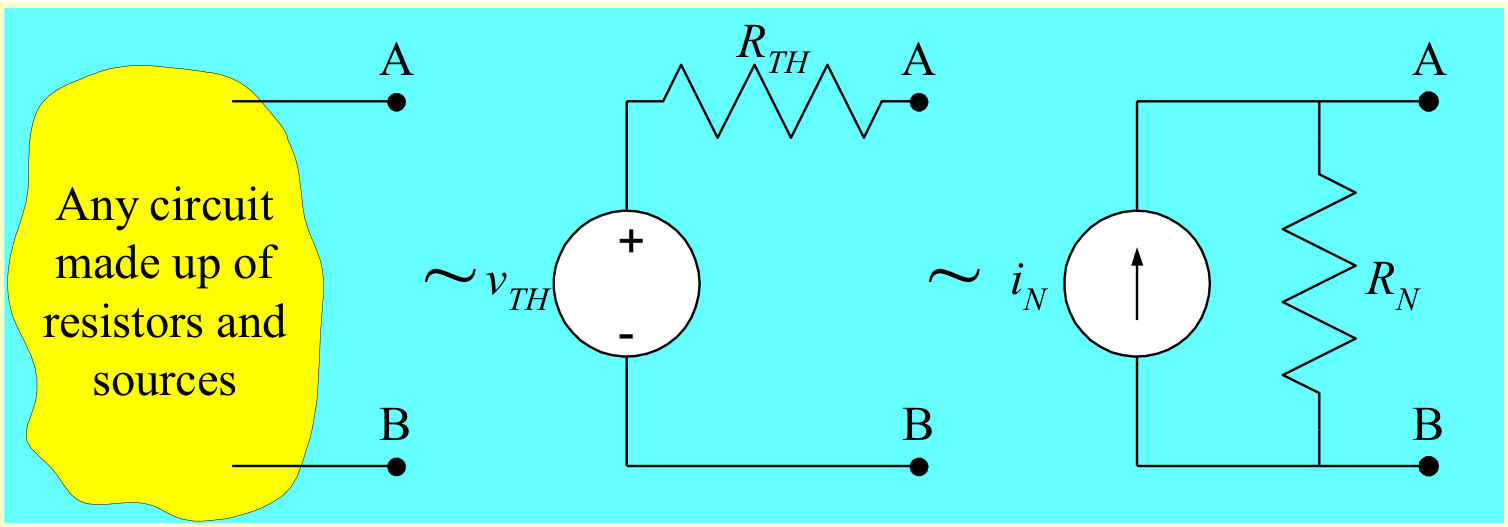Figure 1.25 The Definition of Equivalents. Courtesy of David Shattuck, used with personal permission.

### Equivalent Resistance Reviewed

When we find the equivalent resistance for a Thévenin’s equivalent or a Norton’s equivalent, we set the independent sources equal to zero, and find the equivalent resistance of what remains.

When a dependent source is present, trying to find the equivalent resistance results in a situation we have not dealt with yet. What do we mean by the equivalent resistance of a dependent source?

The answer must be stated carefully. If the ratio of voltage to current for something is a constant, then that something can be said to have an equivalent resistance, since it is behaving as a resistance

### Equivalent Resistance of a Source

So, what we mean by the equivalent resistance of a dependent source is that in this case the ratio of voltage to current is a constant. Then the source can be said to have an equivalent resistance, since it is behaving as a resistance. The equivalent resistance of a dependent source depends on what voltage or current it depends on, and where that voltage or current is in the circuit. It is not easy to predict the answer.This Content is Locked
Only a limited preview of this text is available. You'll need to sign up to Top Hat, and be a verified professor to have full access to view and teach with the content.

### No Equivalent Resistance for an Independent Source

The equivalent resistance of a dependent source, in this case, is the ratio of voltage to current, which is a constant. Then the source can be said to have an equivalent resistance, since it is behaving as a resistance. This will only be meaningful for a dependent source. It is not meaningful to talk about the equivalent resistance of an independent source. The ratio of voltage to current will not be constant for an independent source.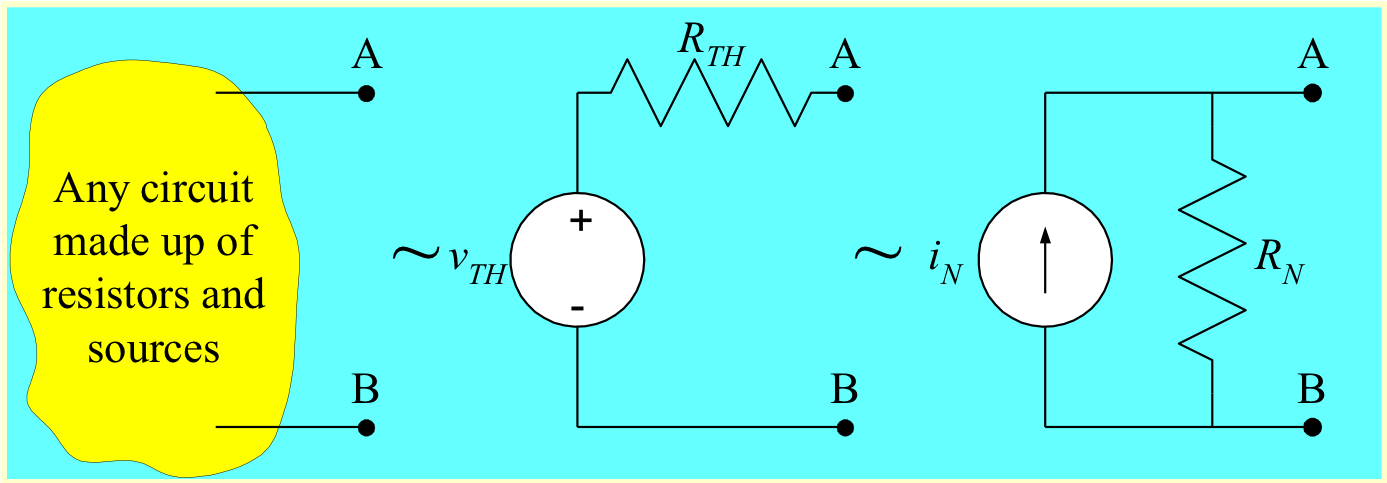Figure 1.25 Repeated Here for Convenience. Courtesy of David Shattuck, used with personal permission.

### Simple Example with a Dependent Source

We will try to explain this by starting with a simple example. We wish to find the equivalent resistance of the circuit below, as seen at terminals A and B.

This will mean that the ratio of the voltage across the circuit, labeled VQ, to the ratio of the current through the circuit, labeled iQ, must be a constant. Let’s find that constant by finding the ratio.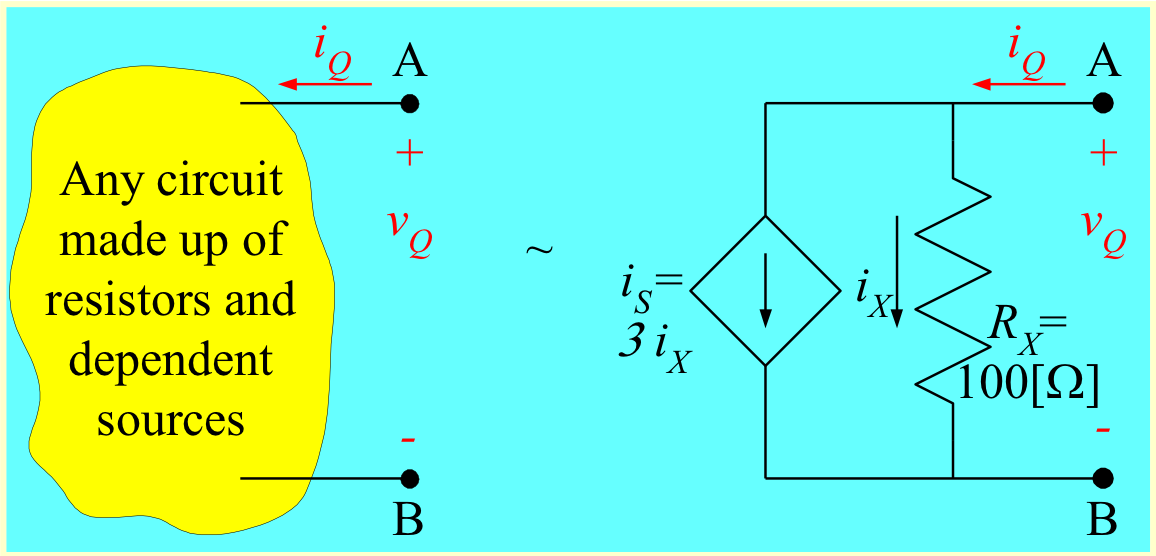Figure 1.26 Simple Example for Equivalent Resistance. Courtesy of David Shattuck, used with personal permission.

We wish to find the equivalent resistance of the circuit above, as seen at terminals A and B.  Let’s find the ratio of the voltage across the circuit, labeled vQ, to the ratio of the current through the circuit, labeled iQ. This must be a constant.  Let’s look first at the circuit equivalent on the right. We note that from Ohm’s Law applied to RX, we can say

Next, we apply KCL at the A node to write that

We take the ratio of them, and plug in the expressions that we found for each. When we do this, we get

Note that ratio is a constant. The ratio has units of resistance, which is what we expect when we take a ratio of a voltage to a current.

The dependent source is in parallel with the resistor RX. Since the parallel combination is 25[$\Omega$], the dependent source must be behaving as if it were a 33.33[$\Omega$] resistor. However, this value depends on RX; in fact, it is RX /3.

### 2nd Simple Example with a Dependent Source

We wish to find the equivalent resistance of a second circuit, given below, as seen at terminals A and B.

Let’s find the ratio of the voltage across the circuit, labeled vQ, to the ratio of the current through the circuit, labeled iQ. This must be a constant. We note that from Ohm’s Law applied to RX, we can say that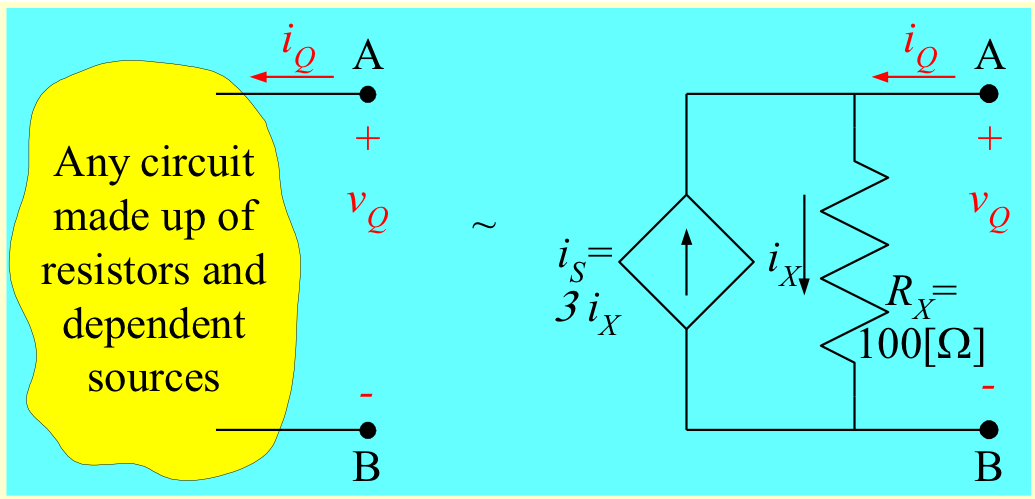Figure 1.27 Circuit for Second Simple Example. Courtesy of David Shattuck, used with personal permission.

Next, we apply KCL at the A node to write that

Note the change in polarity for the source, from the previous example.

We take the ratio of them, and plug in the expressions that we found for each. When we do this, we get

Note that ratio has changed when we simply changed the polarity of the dependent source. The magnitude is not the only thing that changed; the equivalent resistance is now negative

The dependent source is in parallel with the resistor RX. Since the parallel combination is -50[$\Omega$], the dependent source must be behaving as if it were a -33.33[$\Omega$] resistor. This value depends on RX; in fact,  it is  -RX /3.

### Note 1 -- Equivalent Resistance can be Negative

When we find the equivalent resistance for a Thévenin’s equivalent or a Norton’s equivalent, we set the independent sources equal to zero, and find the equivalent resistance of what remains.  We can see that the equivalent resistance can be negative. This is one reason why we have been so careful about polarities all along. We need to get the polarities right to be able to get our signs right.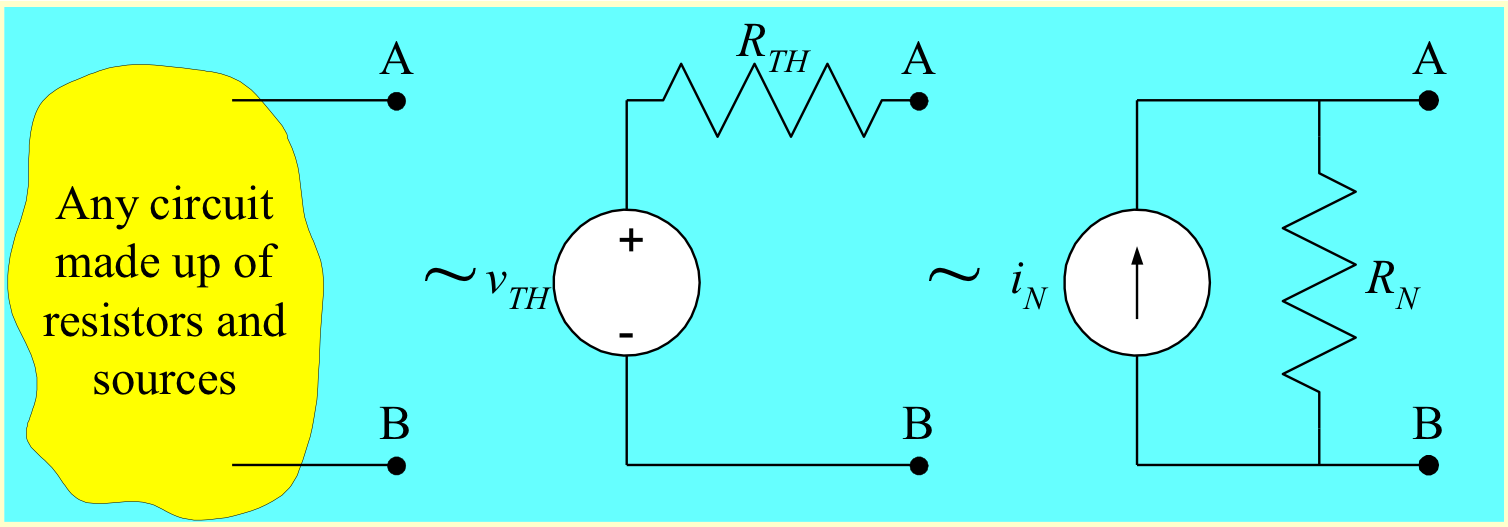Figure 1.25 Repeated for Convenience. Courtesy of David Shattuck, used with personal permission.

### Note 2 -- Test Source Method

In the simple examples that we just did, we were effectively applying a source to the terminals of the circuit. This results in a circuit like others that we have solved before, and we can find the ratio of voltage to current. This is usually easier to think about for most students. It is as if we were applying a source just to test the circuit; we call this method the Test-Source Method.

## Test-Source Method – Defined

To get the equivalent resistance of a circuit, as seen by two terminals of that circuit, we follow these steps.

1. Set all independent sources equal to zero.
2. Find the equivalent resistance.  Do one of the two following bulleted items.
• If there are no dependent sources, find this equivalent resistance using the equivalent resistance rules that have been used before. These include series combinations, parallel combinations, and delta-to-wye equivalents.
• If there are dependent sources present, apply a test source to the two terminals. It can be either a voltage source or a current source.  If you apply a voltage source, find the current through that voltage source.  If you apply a current source, find the voltage across that current source.  Then, find the ratio of the voltage to the current, which will be the equivalent resistance.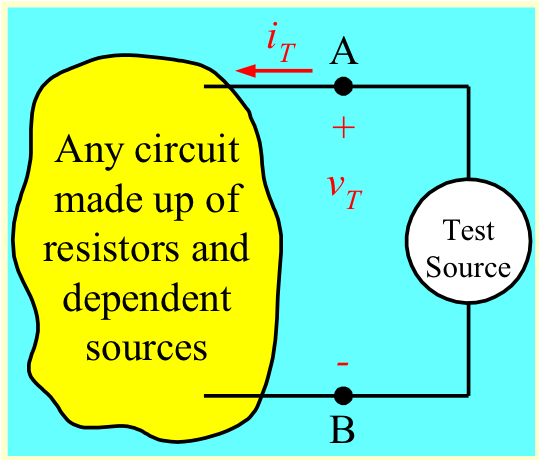Figure 1.28 Test Source Method. Courtesy of David Shattuck, used with personal permission.

### Test-Source Method – Notes

Do not forget step one.  We always set the independent sources to zero.  Some call this "killing" the independent sources.  While this sounds violent, it is important not to forget this step.

In step two, you have two choices.  Pick the easier one, in general.  You do not need to do both.  You could actually pick the second option every time, but the first option is easier. Use it if you can.

When you apply this voltage  and this current, vT and iT, apply them in the active sign relationship for the source. This gives the proper sign; that is, it allows you to write the equation that looks like Ohm's Law with a positive sign.  This actually is Ohm's Law, but it is applied to the circuit made up of resistors and dependent sources, where this voltage and current are in the passive sign relationship.

### Notes

1. The Test-Source Method usually requires some practice before it becomes natural for students. It is important to work several problems to get this practice in.
2. There is a tendency to assume that one could just ignore the Test-Source Method, and just find the open-circuit voltage and short-circuit current whenever a dependent source is present. However, sometimes this does not work. In particular, when the open-circuit voltage and short-circuit current are zero, we must use the Test-Source Method. Learn how to use it.

### Example Problem 1.3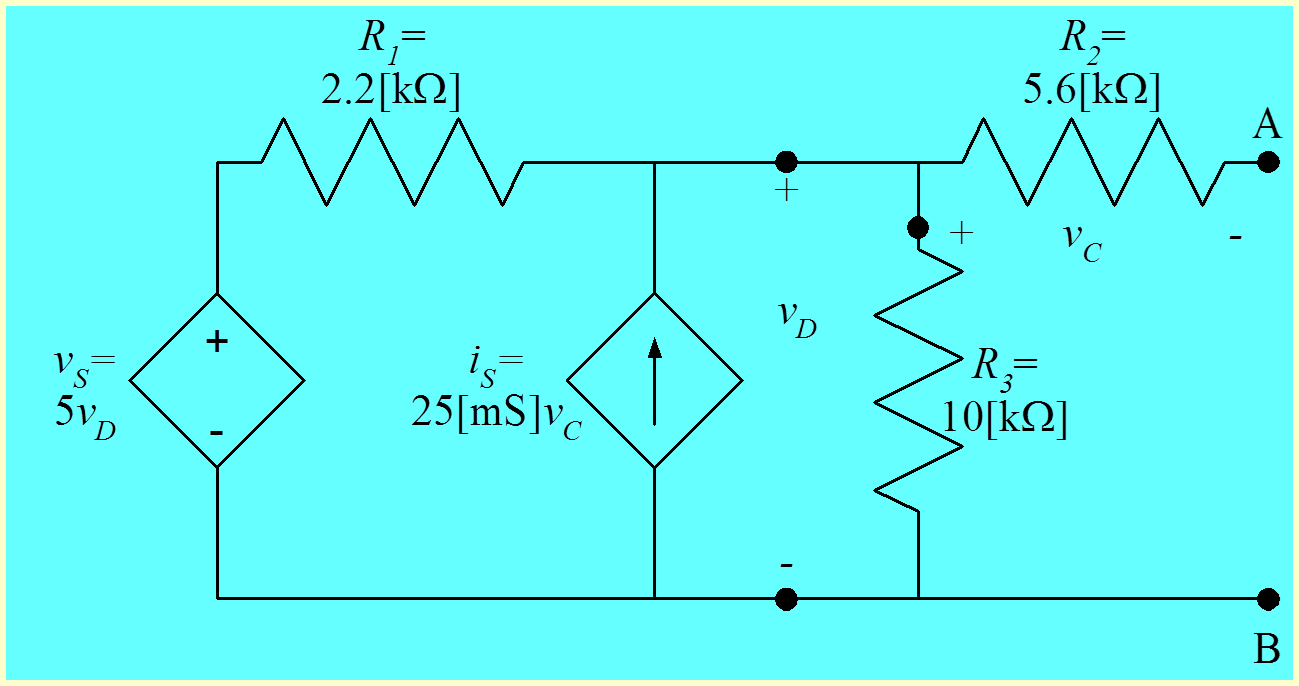Figure 1.29 Circuit for Example Problem 1.3. Courtesy of David Shattuck, used with personal permission.

We wish to find the Thévenin equivalent of the circuit above, as seen from terminals A and B.  We will start by find the open-circuit voltage vOC at those two terminals, as defined below.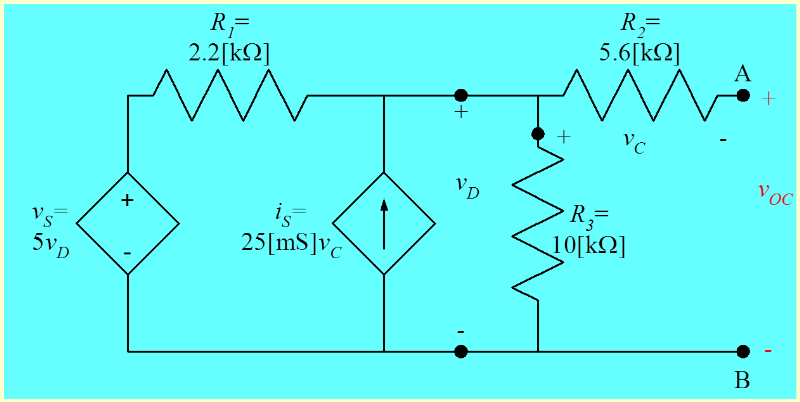Figure 1.30 Defining the Open-Circuit Voltage vOC. Courtesy of David Shattuck, used with personal permission.
Test Source Method

Which component do we know we can ignore when finding vOC?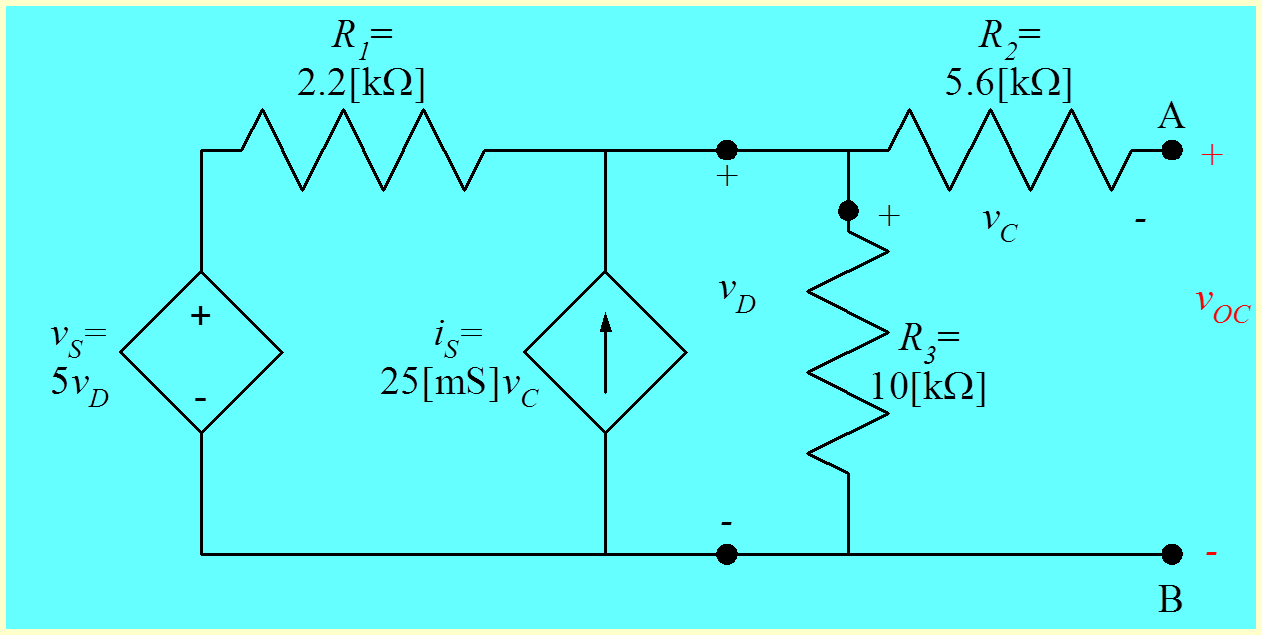To find vOC, we will first find vD, by writing KCL at the top center node. We have

Note that we recognize that the current through R2 must be zero since R2 is in series with an open circuit.  We can substitute in the value for iS, 25[mS]vC.  We note that since the current through R2 is zero, the voltage across it is zero, so vC is zero. So, we write

Next, we substitute in values and solve for vD. We write

Now, we can take KVL around the loop, and we write

The Thévenin voltage is equal to this open-circuit voltage, so the Thévenin voltage must be zero. The short-circuit current will also be zero. To get the resistance, we need to use the Test-Source Method.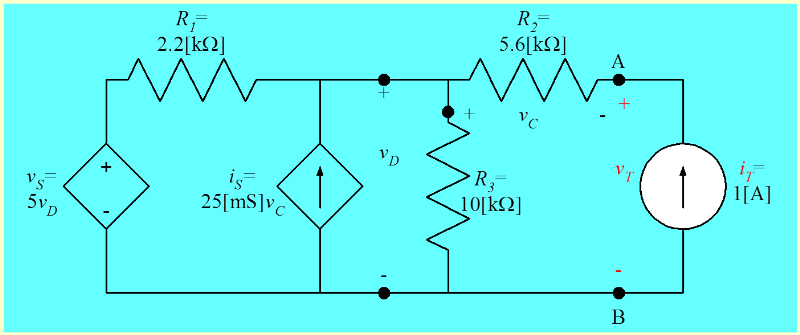Figure 1.31 Applying a Test Source. Courtesy of David Shattuck, used with personal permission.

We have applied a test current source to the two terminals. We have also labeled a voltage across this current source, vT. This voltage has been defined in the active sign relationship for the current source. As noted earlier, this will give us the passive sign relationship for vT and iT for the circuit that we are finding the equivalent resistance of. Thus, we will have

We have applied a test current source to the two terminals. We don’t need to do this, but doing so makes it clear that we are now just solving another circuit, like the many that we have solved before. We have even given the source a value, in this case, 1[A]. This is just a convenience. Many people choose to leave this as an arbitrary source. We choose to use a value, an easy value like 1[A], to allow us to find an actual value for vT.  We have applied a test current source to the two terminals. A test voltage source would have been just as good. We chose a current source because we thought it might make the solution a little easier, since we can find vC so easily now. But it really does not matter. Don’t worry about which one to choose. Let us solve.

Let us solve for vT. We note that we can write an expression for vC using Ohm’s Law, and get

This voltage may seem very large. Don’t let this bother you. We do not actually have this voltage; it is just for calculating the resistance.  Next, let’s write KCL for the top center node. We get

Solving for vD yields

Taking KVL, we get

So, we can find the equivalent resistance by finding

So, the Thévenin equivalent is given in the circuit below. Note that the Thévenin voltage is zero, and so we don’t even show the voltage source at all. The Thévenin resistance is shown, and in this case, it is the Thévenin equivalent.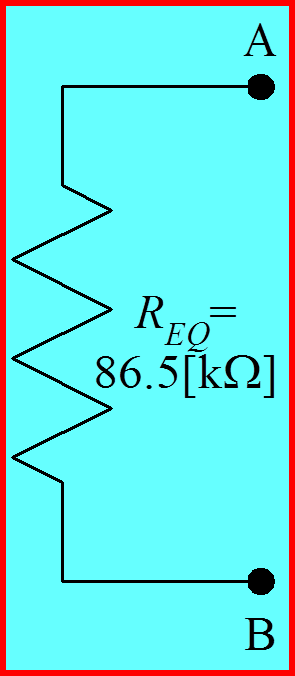Figure 1.32 Solution for Example Problem 1.3. Notice that the solution is only a resistance, since the source is zero. Courtesy of David Shattuck, used with personal permission.

### Summary

Is the Test-Source Method really that important?  This is a good question. Basically, the answer is yes. There are many cases where we have dependent sources present, and wish to use a Thévenin equivalent. While in some cases we can get the Thévenin resistance from the open-circuit voltage and the short-circuit current, there are others where we cannot. The Test-Source Method is also quicker in some cases.  Some students go to great lengths to avoid learning this method. This seems like a waste of energy. Just learn it and use it.

Test Source Method

Should we use a voltage source or a current source when we use the test source method?

A

We must use a voltage source when we have voltage sources in the circuit.

B

We must a voltage source with the Thevenin equivalent, and a current source with the Norton equivalent.

C

We should use the source that makes the solution negative.

D

Yes, we should use a voltage source or a current source.

Sign of the Equivalent Resistance

Will the equivalent resistance always be positive?

A

Yes, because resistance is always positive.

B

No, it will depend on the signs of the independent sources in the circuit.

C

Yes, because positive resistances absorb positive power.

D

No, with dependent sources present, the equivalent resistance can be positive or negative.

Chapter 1 Feedback

Please let us know what you found useful, what you found confusing, and any other comments you might have on Chapter 1. Your responses will be anonymous.

All figures and all equations in this chapter were developed by David Shattuck, University of Houston, Houston, TX, USA.